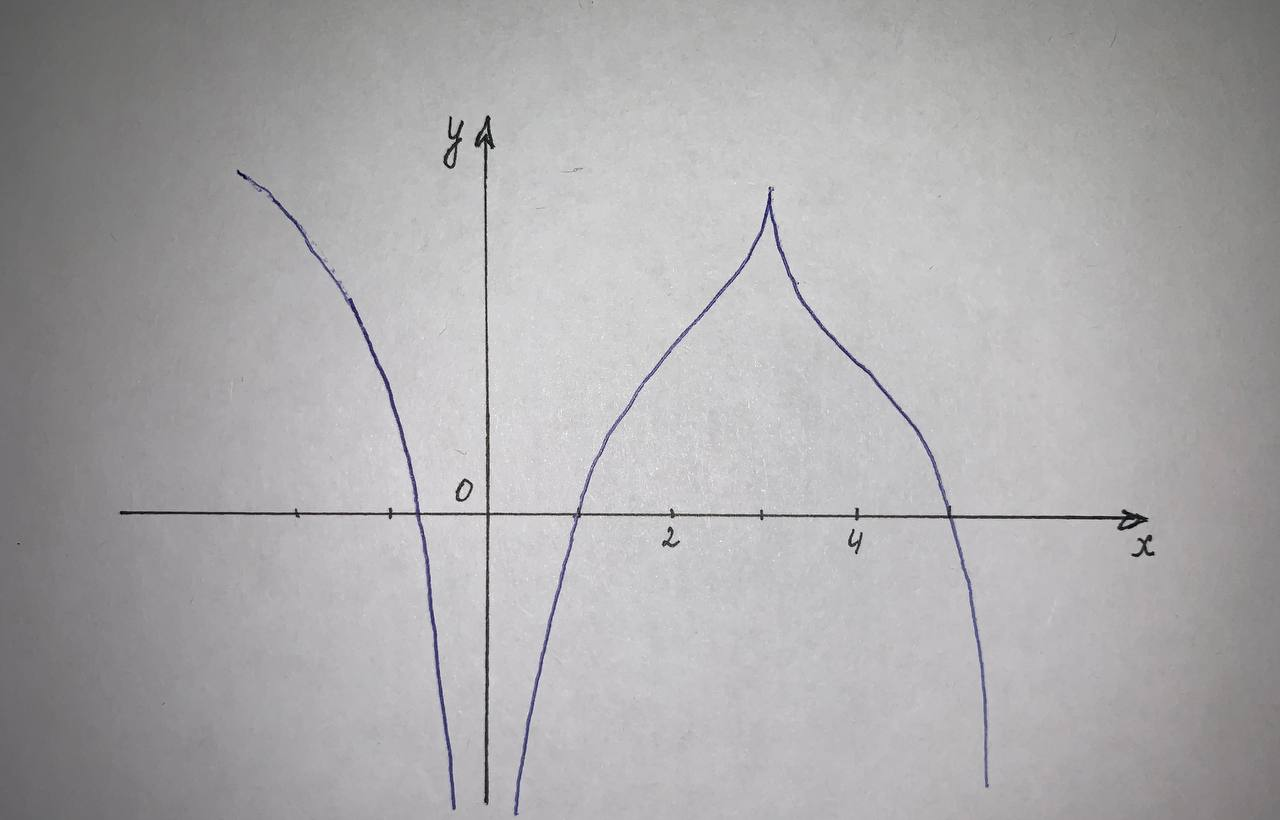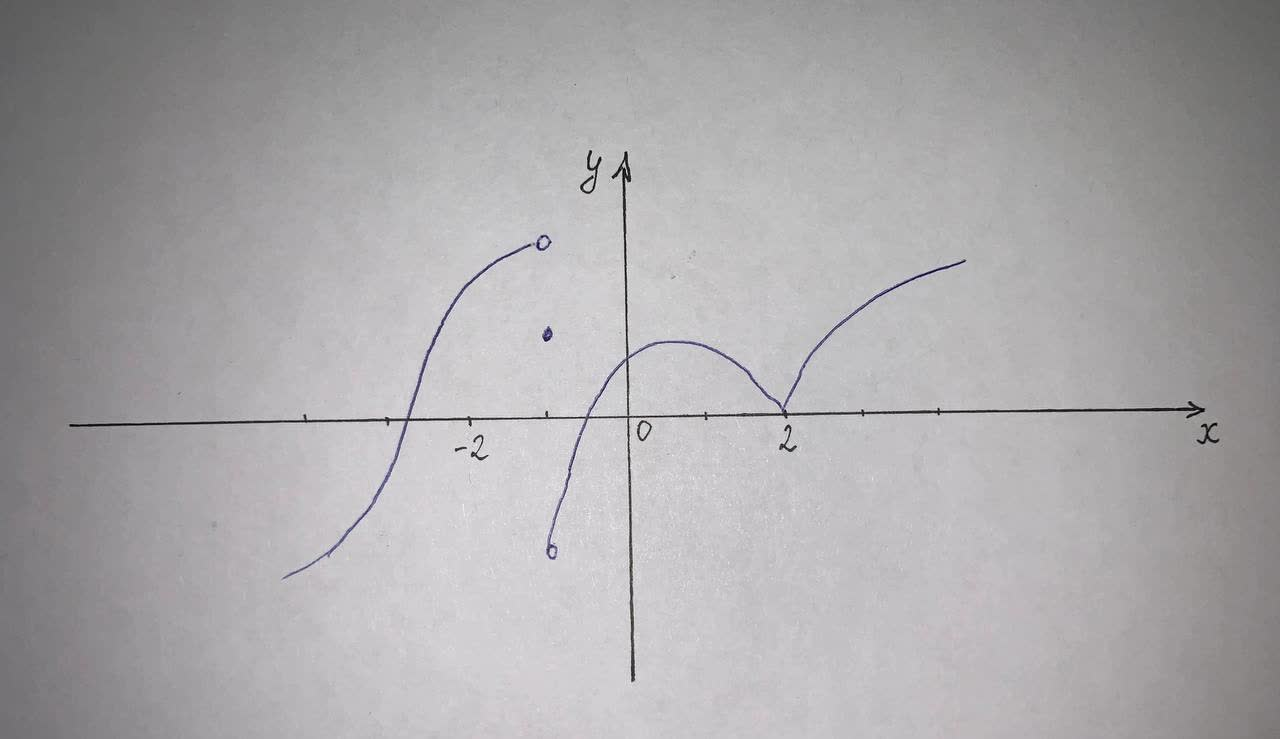# 5)The graph of f is given. State the numbers at which f is not differentiablechillywilly12a 2021-05-13 Answered

5)The graph of f is given. State the numbers at which f is not differentiable.
x=?(smaller value)
x=?(larger value)6)The graph of f is given. State the numbers at which f is not differentiable.
x=?(smaller value)
x=?(larger value)You can still ask an expert for help

• Questions are typically answered in as fast as 30 minutes

Solve your problem for the price of one coffee

• Math expert for every subject
• Pay only if we can solve iti1ziZ